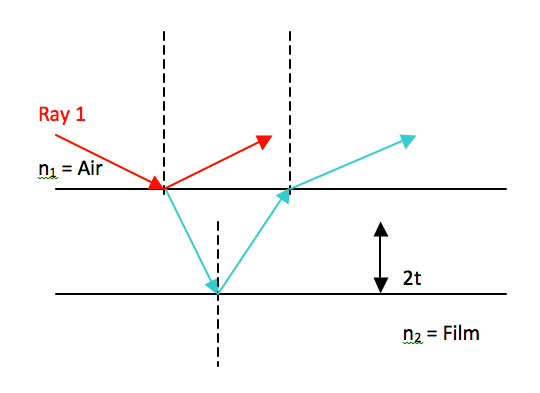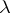# Day 158 physics

Ever wonder why soap bubbles are colored? Ever see some oil in a puddle of water and see different colors on the surface? These phenomena are due to thin film interference.

Basically, when light hits the surface of the liquid, some light is reflected and some is transmitted through the liquid to the bottom surface of the film, where it is then reflected back up to the surface. The interference of light waves at the surface of the film causes the different colors to be seen.

This diagram will help show the interference that can occur at the surface of the film.

Consider air to be the medium above the film, of index of refraction n1, and the film to have an index of refraction n2.• The dashed lines represent normals draw the surface.
• The red lines represent reflection at the surface of the film. Because n2 > n1, there will be a 180o phase change at the surface.
•   If n2 < n1, there will be no phase change upon reflection.
• The aqua wave refracts through the film, reflects off the lower surface and refracts again when re-entering the air. All of the previous reflection and refraction laws are followed here. At the surface there is no phase reversal for this wave.
• 2t represent the vertical distance that the aqua wave must travel through the film and back.

## The Mathematics Behind Thin Film Interference

Since                         n1v1 = n2v2

Then                         n1f11 = n2f22

Since f does not change        n11 = n22

And2= (n11)/n2

Or..n =/n

Where..                                n = index of refraction of the film= wavelength of light in the vacuumn = wavelength of light in the film

If 2t =/2, then Rays 1 and 2 at the surface recombine in phase to give constructive interference (addition of amplitudes).

Or the equation can be used: 2nt = (m +1/2)(m = 0, 1, 2, 3 etc)

Since destructive interference will occur with another wavelength out of phase, the equation for destructive interference is the following:

2nt = m(m=1, 2, 3 etc.)

There are multiple thicknesses of film (depending on the wavelength of the light) that will yield the same results on the surface. That is why there is the factor m in the above equations.

All in all, the two factors that determine the colors and brightness level at the surface of a film are:

• phase reversals on reflection and
• difference in travel distance (t)

(source)